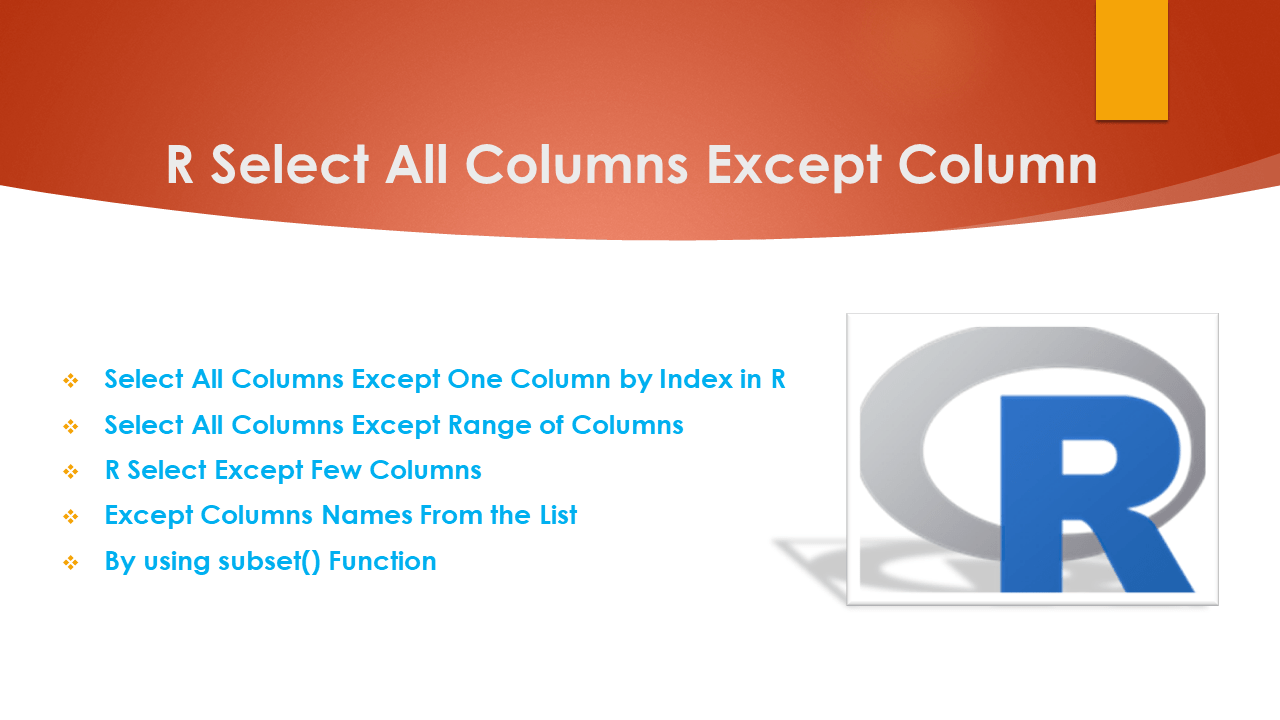# R Select All Columns Except Column

To select all columns except one or a few columns from a data frame in R, you can use the `df[]` notation, `subset()` function, and `select()` function from the `dplyr` package. Below are quick examples. In this article, I will explain how to select all columns except one or a few columns from R Data Frame.

Let’s create an R DataFrame, run these examples and explore the output. If you already have data in CSV you can easily import CSV files to R DataFrame. Also, refer to Import Excel File into R.

``````
# Create dataframe
df=data.frame(id=c(11,22),
pages=c(32,45),
name=c("spark","python"),
chapters=c(76,86),
price=c(144,553))

# Display the dataframe
print(df)

# Output
#  id pages   name chapters price
#1 11    32  spark       76   144
#2 22    45 python       86   553
``````

## 1. Select All Columns Except One Column by Index in R

First, let’s use the R base bracket notation `df[]` to select all columns except one column by Index. This notation takes syntax `df[, columns]` to select columns in R, And to ignore columns you have to use the – (negative) operator.

The following example selects all columns from the Data frame except the second column from the R DataFrame.

``````
# Select All Columns Except Second column
df2 <- df[,-2]
df2

# Output
id   name chapters price
1 11  spark       76   144
2 22 python       86   553
``````

## 2. Select All Columns Except Range of Columns

This notation also supports selecting all columns except the range of columns. In the following example, selects all columns except columns between `2` and `4` indexes.

``````
# select except range of columns
df2 <- df[,-2:-4]
df2

# Output
id price
1 11   144
2 22   553
``````

## 3. R Select Except Few Columns

Use vector to specify the column/vector indexes you want to ignore the columns. The following example removes multiple columns with indexes 2 and 3.

``````
# Except Multiple columns
df2 <- df[,-c(2,3)]
df2

# Output
id chapters price
1 11       76   144
2 22       86   553
``````

### 4. Except Columns Names From the List

You can also use the column names from the list to ignore them from the R data frame. Here I am using names() function which returns all column names and checks if a name is present in the list using %in% operator.

``````
# Except Columns in List
df2 <- df[,!names(df) %in% c("id", "name", "chapters")]
df2

# Output
pages price
1    32   144
2    45   553
``````

### 5. By using subset() Function

By using the R base function subset() you can select columns except specific columns from the data frame. This function takes the data frame object as an argument and the columns you wanted to remove.

``````
#using subset
df2 <- subset(df, select = -c(id, name, chapters))
df2

``````

Yields the same output as above.

## 6. R Select Columns Except Column By using dplyr Functions

In this section, I will use functions from the dplyr package to select all columns except specific columns in R data frame. dplyr is an R package that provides a grammar of data manipulation and provides a most used set of verbs that helps data science analysts to solve the most common data manipulation. In order to use this, you have to install it first using `install.packages('dplyr')` and load it using `library(dplyr)`.

dplyr select() function is used to select the column and by using negation of this to ignore columns.

``````
library("dplyr")

# selectall except columns using select()
df2 <- df %>% select(-c(id, name, chapters))

# Output
pages price
1    32   144
2    45   553
``````

## 7. Complete Example

The following is a complete example of how to select all columns except one or a few columns from the R DataFrame (data.frame)

``````
# Create dataframe
df=data.frame(id=c(11,22,33,44,55),
pages=c(32,45,33,22,56),
name=c("spark","python","R","java","jsp"),
chapters=c(76,86,11,15,7),
price=c(144,553,321,567,890))

# Display the dataframe
print(df)

# select Columns except by Index
df2 <- df[,-2]

# select Columns by except Range of columns
df2 <- df[,-2:-4]

# select all except Multiple columns
df2 <- df[,-c(2,3)]

# except Columns in List
df2 <- df[,!names(df) %in% c("id", "name", "chapters")]

# using subset
df2 <- subset(df, select = -c(id, name, chapters))

library("dplyr")

# using select()
df2 <- df %>% select(-c(id, name, chapters))
``````

## Conclusion

In this article, you have learned different ways to select all columns except a few columns from the R data frame. The example includes ignoring columns by name, index, from the list based on conditions e.t.c. Also learned how to use a select() function from the dplyr package.

## Related Articles

### Naveen (NNK)

Naveen (NNK) is a Data Engineer with 20+ years of experience in transforming data into actionable insights. Over the years, He has honed his expertise in designing, implementing, and maintaining data pipelines with frameworks like Apache Spark, PySpark, Pandas, R, Hive and Machine Learning. Naveen journey in the field of data engineering has been a continuous learning, innovation, and a strong commitment to data integrity. In this blog, he shares his experiences with the data as he come across. Follow Naveen @ @ LinkedIn